# Exact Values: Simplifying surds

Any time we meet an irrational number in our work, we have the choice of decimalizing the number or leaving it in symbolic form.

The symbolic form of a number is the notation we use to capture the meaning of the number. For example,As can be seen from some of these ‘meanings’ the symbolic form captures a lot of information in a small amount of space on paper. There are also sometimes more than one meaning, or more than one equivalent definition.

When we decimalize, we get an idea of the magnitude of the number. Eg,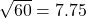(2 d.p) This can be very useful, especially if plotting the value on a graph. However, the downside is that we lose accuracy. Rounded decimals are  not ‘exact answers’.

When we keep the number in symbolic form we maintain accuracy. Irrationals given in symbolic form, (along with any other non-rounded form of number such as fractions, integers or terminating decimals), are exact values.

# Simplifying a surd

A surd is the square root of a non-square number and shows up often when using the quadratic formula. It is irrational, and can often be simplified.

First, you need to factor the number under the square root (radicand). We’re looking for the highest factor that is a perfect square.

The first 12 positive square numbers are: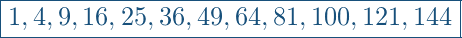Example: SimplifyLet’s list the factors of 72.

Here are the factors: 1, 2, 3, 4, 6, 8, 9, 12, 18, 24, 36, 72

The factors that are also perfect squares are: 4, 9, 36 (also 1 but 1 is not useful here).

The highest is 36.

So,Suppose I selected 9 or 4 because I didn’t spot that 36 was a factor. In this case, I end up with another surd that can be simplified, so I just simplify again.

e.g.andTherefore,Simplify the square roots by writing as a product of a square number with a non square number:

# Simplifying solutions to a quadratic equation

Let’s apply this method of simplification to the solutions of a quadratic equation. Use the quadratic formula to solve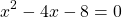Substitute these values to the formula we have:From this we can conclude that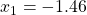,(2 d.p)

However, we can also simplify the exact value of this answer as follows:Replacewith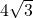in the solutions:Similarly,.

We can check on a calculator thatand.

The exact answers for this equation areand.